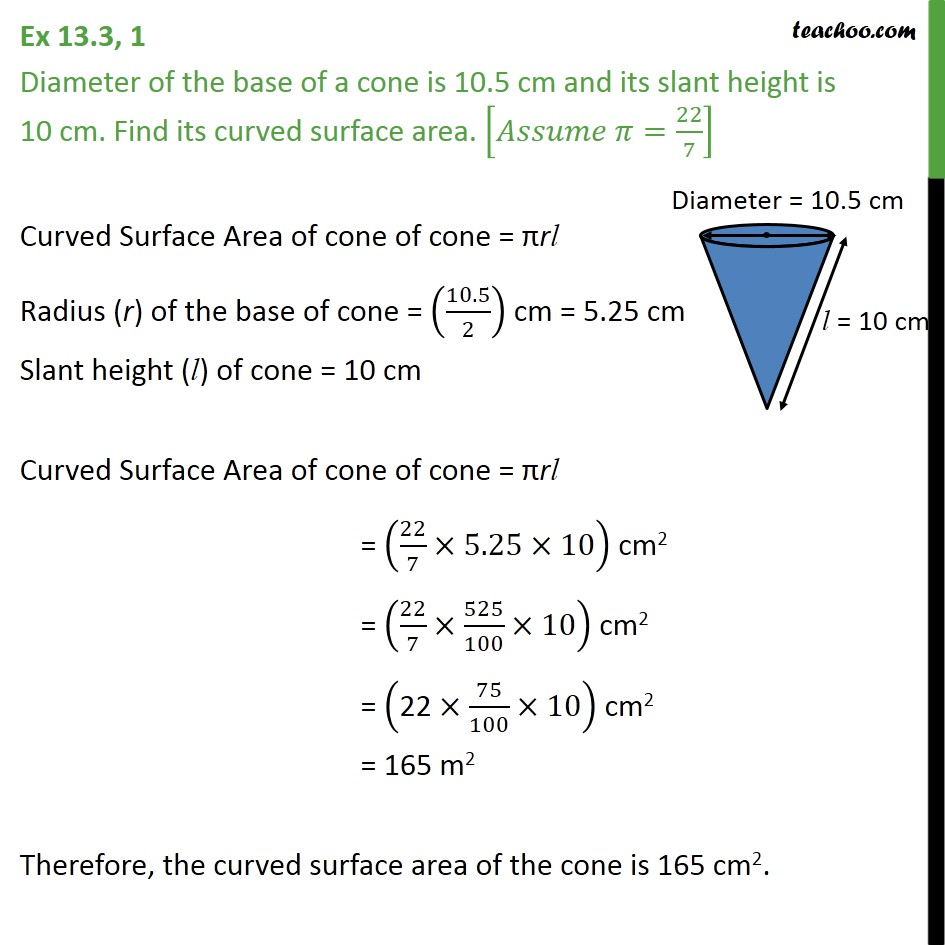Ex 11.1

Chapter 11 Class 9 Surface Areas and Volumes
Serial order wiseLearn in your speed, with individual attention - Teachoo Maths 1-on-1 Class

### Transcript

Ex 11.1, 1 Diameter of the base of a cone is 10.5 cm and its slant height is 10 cm. Find its curved surface area. [𝐴𝑠𝑠𝑢𝑚𝑒 𝜋=22/7] Curved Surface Area of cone of cone = πrl Radius (r) of the base of cone = (10.5/2) cm = 5.25 cm Slant height (l) of cone = 10 cm Curved Surface Area of cone of cone = πrl = (22/7×5.25×10) cm2 = (22/7×525/100×10) cm2 = ("22" ×75/100×10) cm2 = 165 m2 Therefore, the curved surface area of the cone is 165 cm2.